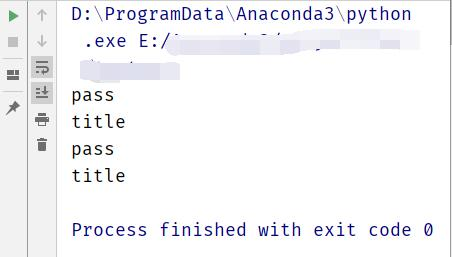Python for循环和if判断问题
``````if cc['filter']:
for key,value in cc['filter'].items():
for cs in value:
if re.search(cs,item[key]) is None:
print('pass')
item = item
else:
print('not pass')
item = None
break
return item
``````

``````'filter':{
'title':
[
'.*?_国网',
'题材瞻',
],
'content':
[
'\(来源：.*?\)'
],
'source':[
'.*?hangq'
],
'url':[]
}`

``````

item的数据：

``````
item = {'content': '(来源：新浪网)一、题材前瞻 1、MicroLED显示技术突破量产关卡商用进程有望进一步加速、国星光电、华灿光电。 (来源：中国网财经)',
'source': '旺角资讯',
'title': '个股精选(题材前瞻)_中国网',
'url': '',
}
``````1个回答8 个月之前 回复huifaguang 应该是的吧，不然会报错呀
8 个月之前 回复python怎么用循环删除列表中的重复数值
a=[1,2,1,4,1,] n=a while n<len(a)+1: if a[n]==1: a.remove(a[n]) n=n+1 print (a) 这样写执行的结果列表最后的1删不掉 a=[1,2,1,4,1,] n=a while n<len(a)+1: if a[n]==1: del a[n] n=n+1 print (a) 判断条件下的删除改成del,最前面的1删不掉。 a=[1,2,1,4,1,] n=a while n<len(a)+1: if a[n]==1: a.pop(n) n=n+1 print (a) 把判断条件下的删除改成pop,最前面的1也是删不掉 a=[1,2,1,4,1,] for i in a: if i==1: a.remove(1) print (a) 改成for循环，又没问题 现在有个问题，就是删除重复值1，for循环配合del,pop,remove怎么写，while循环配合del,pop,remove代码又该怎么写。可能我的问题有些复杂。请原谅我是小白。希望大神不吝赐教。谢谢
python如何在正常退出和满足某个条件退出循环，执行不同代码
``` #例如 a=[0,1,2,3,4],判断某一元素是不是在a中，（只是举例） b=4 for item in a: if(b==item)； break #找到和没找到有不同输出,如何让程序自己判断是哪种退出循环 print("yes") print("no") ```

python怎样改变循环次数（小白求）
for i in range(0,6): print i i = i+1 输出结果是： 0 1 2 3 4 5 如果我只想输出 0 2 4 该怎么做？不要用If语句判断。

![图片说明](https://img-ask.csdn.net/upload/201910/11/1570764654_226893.png) ``` import os lst = os.listdir(os.getcwd()) # 获取当前目录下所有的文件名 for c in lst: if os.path.isfile(c) and c.endswith('.py') and c.find("run")== -1: #判断文件名是以.py结尾的，并且去掉run.py文件 print(c) #查看文件 os.system('python {}'.format(c)) #相当于在终端执行文件 python main.py ``` 用这段代码的问题就是，第一个0.py文件运行后，因为是一个死循环的程序，所以后面的文件一直不能运行，而且后面的文件也是死循环的文件。
python3 word="0"的编码如何变为“utf-8”
python3 最近要通过python实现搜索文件中的关键词出现次数的功能，定义输入的关键字字符串为word=""，代码从docx文件读取编码为"utf-8"，然后进行匹配搜索。目前输入word="1",word="0"都会报错，word="1234"就不会报错，分析大概是**编码问题**导致的。总的来说，我希望检索“0”这个字符串在某个word文档中出现的次数；是需要从word加载的内容全部转为unicode或者utf-8编码再匹配查找吗？目前“0”会在循环的某个判断停掉 ：if i.find(word) != -1:，关于在匹配关键词时用什么编码这块比较小白，希望大神可以帮忙看下： ``` # -*- coding: UTF-8 -*- from docx import Document import re, chardet filename = "D:\python测试\科目四.docx" word = "米".encode(encoding='utf-8') #打开文档 document = Document(filename) print (filename) #读取每段资料 l = [paragraph.text.encode(encoding='utf-8', errors='ignore') for paragraph in document.paragraphs] count = 0 count_2 = 0 j = 0 for i in l: i = i.strip() if i.find(word) != -1: count = count + 1 j = j + 1 print('-', count, '-', i.decode('utf-8')) print("计数： ", j) count_2 = count_2 + j print("该篇中出现字符的个数为:", count_2) ``` 每次报错不一样，有时就是直接循环结束但最后一个print没有执行，也没有任何报错，和输入word参数有关: ![图片说明](https://img-ask.csdn.net/upload/201909/25/1569399847_808735.jpg) 如果把编码全部去掉，大部分输入没问题，但是当word="0"时最后一个print没输出，这个如何解释 ############################ 刚才又改了下，如果加try就会运行正确，不加try就不打印最后一个print： ![图片说明](https://img-ask.csdn.net/upload/201909/25/1569404197_644224.jpg) 如下是正确的输出： ![图片说明](https://img-ask.csdn.net/upload/201909/25/1569404323_410334.jpg)

python3 用pdfminer3k爬取PDF文件不完整，请问有什么解决方法吗？

pygame.error: video system not initialized

Python 3.5代码求助，卡在截取数字的步骤上

Python绘制词频统计图，怎么输出到GUI，而不是输出到控制台？
# -*- coding: utf-8 -*- from tkinter import * import tkinter import hashlib import time import codecs import codecs import matplotlib.pyplot as plt from pylab import mpl mpl.rcParams['font.sans-serif'] = ['FangSong'] # 指定默认字体 mpl.rcParams['axes.unicode_minus'] = False # 解决保存图像是负号'-'显示为方块的问题 LOG_LINE_NUM = 0 class MY_GUI(): def __init__(self,init_window_name): self.init_window_name = init_window_name #设置窗口 def set_init_window(self): self.init_window_name.title("维吉尼亚密码工具") #窗口名 self.init_window_name.geometry('320x160+10+10') #290 160为窗口大小，+10 +10 定义窗口弹出时的默认展示位置 self.init_window_name.geometry('1000x680+10+10') self.init_window_name["bg"] = "white" #窗口背景色，其他背景色见：blog.csdn.net/chl0000/article/details/7657887 self.init_window_name.attributes("-alpha",1.0) #虚化，值越小虚化程度越高 self.init_data_label = Label(self.init_window_name, text="待处理数据") self.init_data_label.grid(row=0, column=0) self.result_data_label = Label(self.init_window_name, text="输出结果") self.result_data_label.grid(row=0, column=15) self.init_key_label = Label(self.init_window_name, text="密钥") self.init_key_label.grid(row=0, column=10) self.log_label = Label(self.init_window_name, text="日志") self.log_label.grid(row=12, column=0) self.init_data_Text = Text(self.init_window_name, width=50, height=35) # 原始数据录入框 self.init_data_Text.grid(row=1, column=0, rowspan=10, columnspan=10) self.result_data_Text = Text(self.init_window_name, width=35, height=49) # 处理结果展示 self.result_data_Text.grid(row=1, column=12, rowspan=15, columnspan=10) self.key_Text = Text(self.init_window_name, width=5, height=1) # 密钥框 self.key_Text.grid(row=1, column=6, rowspan=1,columnspan=10) self.log_data_Text = Text(self.init_window_name, width=66, height=9) # 日志框 self.log_data_Text.grid(row=13, column=0, columnspan=10) #按钮 self.str_transtoencrypt_button = Button(self.init_window_name, text="加密", bg="lightblue", width=10,command=self.str_transtoencrypt) # 调用内部方法 加()为直接调用 self.str_transtoencrypt_button.grid(row=3, column=11) self.str_transtodecrypt_button = Button(self.init_window_name, text="解密", bg="lightblue", width=10,command=self.str_transtodecrypt) # 调用内部方法 加()为直接调用 self.str_transtodecrypt_button.grid(row=5, column=11) self.str_count_button = Button(self.init_window_name, text="词频统计", bg="lightblue", width=10,command=self.str_count) # 调用内部方法 加()为直接调用 self.str_count_button.grid(row=7, column=11) #功能算法的实现 #加密算法 def str_transtoencrypt(self): letter_list = 'ABCDEFGHIJKLMNOPQRSTUVWXYZ'; src = self.init_data_Text.get(1.0,END).strip().replace("\n","") #获得输入的字符 key = self.key_Text.get(1.0, END).strip().replace("\n", "") #获得输入的密钥 a=[] for ch in key: a.append(ord(ch.upper())-65) #ord()函数返回ASCii值 upper()将小写转大写Python 列表 append() 方法用于在列表末尾追加新的对象。 get_list = a ciphertext = ''; i = 0 for ch in src: # 遍历明文 if i % len(get_list) == 0: i = 0 if ch.isupper(): # isupper函数检测字符串中是否都是大写字母 ciphertext += letter_list[(ord(ch) - 65 + get_list[i]) % 26] i += 1 else: ciphertext += letter_list[(ord(ch) - 97 + get_list[i]) % 26].lower() i += 1 self.result_data_Text.delete(1.0, END) self.result_data_Text.insert(1.0, ciphertext) #解密算法 def str_transtodecrypt(self): letter_list = 'ABCDEFGHIJKLMNOPQRSTUVWXYZ'; src = self.init_data_Text.get(1.0, END).strip().replace("\n", "") # 获得输入的字符 key = self.key_Text.get(1.0, END).strip().replace("\n", "") # 获得输入的密钥 a=[] for ch in key: a.append(ord(ch.upper())-65) #ord()函数返回ASCii值 upper()将小写转大写Python 列表 append() 方法用于在列表末尾追加新的对象。 get_list = a ciphertext = ''; i = 0 plaintext=''; i = 0 for ch in src: #遍历密文 if i%len(get_list)==0: i=0 #判断是否是大写 if ch.isupper(): plaintext+=letter_list[(ord(ch)-65-get_list[i]) % 26] i+=1 else: plaintext+=letter_list[(ord(ch)-97-get_list[i]) % 26].lower() i+=1 self.result_data_Text.delete(1.0, END) self.result_data_Text.insert(1.0, plaintext) #词频统计及统计图 def str_count(self): top = Toplevel() top.title('词频统计结果') top.geometry('1000x1000') fr =self.init_data_Text.get(1.0,END).strip().replace("\n","") word = [] counter = {} for line in fr: line = line.strip() if len(line) == 0: continue for w in line: if not w in word: word.append(w) if not w in counter: counter[w] = 0 else: counter[w] += 1 counter_list = sorted(counter.items(), key=lambda x: x, reverse=True) print(counter_list[:50]) label = list(map(lambda x: x, counter_list[:50])) value = list(map(lambda y: y, counter_list[:50])) plt.bar(range(len(value)), value, tick_label=label) plt.show() def gui_start(): init_window = Tk() #实例化出一个父窗口 RMC_PORTAL = MY_GUI(init_window) # 设置根窗口默认属性 RMC_PORTAL.set_init_window() init_window.mainloop() #父窗口进入事件循环，可以理解为保持窗口运行，否则界面不展示 gui_start() ``` ``` ![如图，点击词频统计按钮后，统计图出现在IDE中，同时生成一个空白窗口图片说明](https://img-ask.csdn.net/upload/201810/12/1539334357_812708.jpg)

Java学习的正确打开方式

Spring Boot2 系列教程(十七)SpringBoot 整合 Swagger2

Python 基础（一）：入门必备知识

Python十大装B语法
Python 是一种代表简单思想的语言，其语法相对简单，很容易上手。不过，如果就此小视 Python 语法的精妙和深邃，那就大错特错了。本文精心筛选了最能展现 Python 语法之精妙的十个知识点，并附上详细的实例代码。如能在实战中融会贯通、灵活使用，必将使代码更为精炼、高效，同时也会极大提升代码B格，使之看上去更老练，读起来更优雅。 1. for - else 什么？不是 if 和 else 才

2019年11月中国大陆编程语言排行榜
2019年11月2日，我统计了某招聘网站，获得有效程序员招聘数据9万条。针对招聘信息，提取编程语言关键字，并统计如下： 编程语言比例 rank pl_ percentage 1 java 33.62% 2 c/c++ 16.42% 3 c_sharp 12.82% 4 javascript 12.31% 5 python 7.93% 6 go 7.25% 7

2020 网络课 智慧树自动刷课代码，自动跳转，自动答题并关闭弹窗，自动1.5倍速静音

SQL-小白最佳入门sql查询一

“狗屁不通文章生成器”登顶GitHub热榜，分分钟写出万字形式主义大作

IT界知名的程序员曾说：对于那些月薪三万以下，自称IT工程师的码农们，其实我们从来没有把他们归为我们IT工程师的队伍。他们虽然总是以IT工程师自居，但只是他们一厢情愿罢了。 此话一出，不知激起了多少(码农)程序员的愤怒，却又无可奈何，于是码农问程序员。 码农：你知道get和post请求到底有什么区别？ 程序员：你看这篇就知道了。 码农：你月薪三万了？ 程序员：嗯。 码农：你是怎么做到的? 程序员：

《程序人生》系列-这个程序员只用了20行代码就拿了冠军

8年经验面试官详解 Java 面试秘诀
作者 | 胡书敏 责编 | 刘静 出品 | CSDN（ID：CSDNnews） 本人目前在一家知名外企担任架构师，而且最近八年来，在多家外企和互联网公司担任Java技术面试官，前后累计面试了有两三百位候选人。在本文里，就将结合本人的面试经验，针对Java初学者、Java初级开发和Java开发，给出若干准备简历和准备面试的建议。   Java程序员准备和投递简历的实

1.两种思维方式在求职面试中，经常会考察这种问题：北京有多少量特斯拉汽车？ 某胡同口的煎饼摊一年能卖出多少个煎饼？ 深圳有多少个产品经理？ 一辆公交车里能装下多少个乒乓球？ 一

so easy！ 10行代码写个"狗屁不通"文章生成器